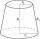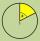# Cone A2V

Surface of cone in the plane is a circular arc with central angle of 126° and area 415 cm2.

Calculate the volume of a cone.

Result

V =  881.1 cm3

#### Solution:Leave us a comment of example and its solution (i.e. if it is still somewhat unclear...):

Showing 0 comments:Be the first to comment!#### To solve this verbal math problem are needed these knowledge from mathematics:

Need help calculate sum, simplify or multiply fractions? Try our fraction calculator. Pythagorean theorem is the base for the right triangle calculator.

## Next similar examples:

1. Cut and coneCalculate the volume of the rotation cone which lateral surface is circle arc with radius 15 cm and central angle 63 degrees.
2. Castle towerThe castle tower has a cone-shaped roof with a diameter of 10 meters and a height of 8 meters. Calculate how much m² of coverage is needed to cover it if we must add one-third for the overlap.
3. Truncated cone 3The surface of the truncated rotating cone S = 7697 meters square, the substructure diameter is 56m and 42m, determine the height of the tang.
4. Cone containerRotary cone-shaped container has a volume 1000 cubic cm and a height 12 cm. Calculate how much metal we need for making this package.
5. Cone 15The radius of the base of a right circular cone is 14 inches and it's height 18 inches. What is the slant height?
6. Truncated cone 5The height of a cone 7 cm and the length of side is 10 cm and the lower radius is 3cm. What could the possible answer for the upper radius of truncated cone?
7. Slant heightThe slant height of cone is 5cm and the radius of its base is 3cm, find the volume of the cone
8. DiscCircumference of the disk is 78.5 cm. What is the circumference of the circular arc of 32° on the disc?
9. Chord - TS v2The radius of circle k measures 87 cm. Chord GH = 22 cm. What is TS?
10. Common chordTwo circles with radius 17 cm and 20 cm are intersect at two points. Its common chord is long 27 cm. What is the distance of the centers of these circles?
11. Reference angleFind the reference angle of each angle:
12. Circle's chordsIn the circle there are two chord length 30 and 34 cm. The shorter one is from the center twice than longer chord. Determine the radius of the circle.
13. ChordIt is given to a circle k(r=6 cm) and the points A, B such that / AB / = 8 cm lies on k. Calculate the distance of the center of circle S to the midpoint C of the segment AB.
14. Theorem proveWe want to prove the sentence: If the natural number n is divisible by six, then n is divisible by three. From what assumption we started?
15. TetrahedronCalculate height and volume of a regular tetrahedron whose edge has a length 18 cm.
16. Circle annulusThere are 2 concentric circles in the figure. Chord of larger circle 10 cm long is tangent to the smaller circle. What are does annulus have?
17. Holidays - on poolChildren's tickets to the swimming pool stands x € for an adult is € 2 more expensive. There was m children in the swimming pool and adults three times less. How many euros make treasurer for pool entry?